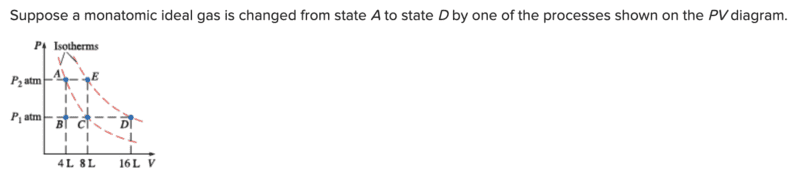# Monatomic ideal gas question

hockeyfan123
Homework Statement:
Monoatomic ideal gas question
Relevant Equations:
Internal EnergyP1=1.9 and P2=4.8.

Question: what is the total change in internal energy

This is what I have so far but it is still incorrect I believe:

U= (3/2)(1/2)(2.9)(1.01x10^3)(8x10^-3)

Where am I going wrong?

Homework Helper
Gold Member
Homework Statement:: Monoatomic ideal gas question
Relevant Equations:: Internal Energy

View attachment 318195

P1=1.9 and P2=4.8.

Question: what is the total change in internal energy

This is what I have so far but it is still incorrect I believe:

U= (3/2)(1/2)(2.9)(1.01x10^3)(8x10^-3)

Where am I going wrong?
Hello and welcome to PF!

It's not clear what formula you are using. You did not list the formula in the "Relevant Equations" section. Also, it would be good to include the units with your numerical values.

It appears to me that the data given in the problem is inconsistent. The change in internal energy should not depend on the particular path going from state A to state D. But I find different changes in energy for different paths.

Homework Helper
Gold Member
I don't quite understand this equation
U= (3/2)(1/2)(2.9)(1.01x10^3)(8x10^-3)
Please write symbolically first then show the numbers that you substitute. You can post equations in LateX. Click on the link "LaTeX guide", lower left, to learn how.

One wrong thing is that your answer is an internal energy at some point, not clear which. You are supposed to find the change in internal energy from A to D. This means the difference ##U_D-U_A##.

Homework Helper
Gold Member
Another way to see that the data is inconsistent is to consider states E and D. They are at the same temperature. State E has half the volume of state D. Therefore, from Boyles's law (or from PV = nRT), state E should have twice the pressure of state D. But ##P_D## is given to be 1.9 atm and ##P_E## is given to be 4.8 atm. Check to see if the 4.8 atm was actually given as 3.8 atm.

Mentor
The two equations you should be working with are $$\Delta U=nC_v\Delta T$$ and $$\Delta (PV)=nR\Delta T$$